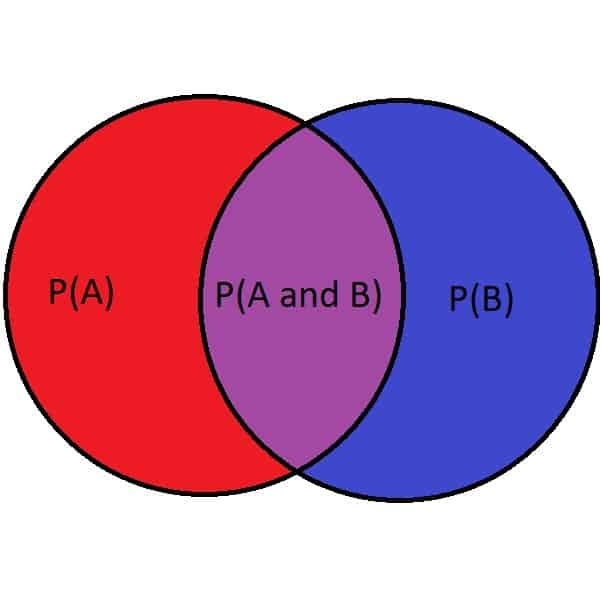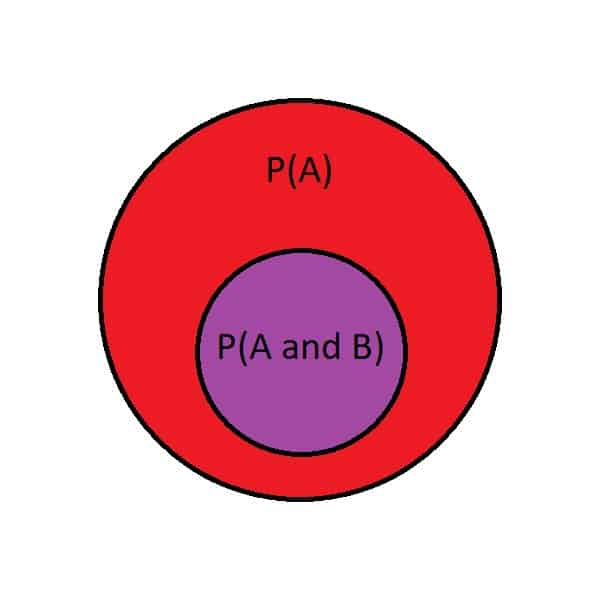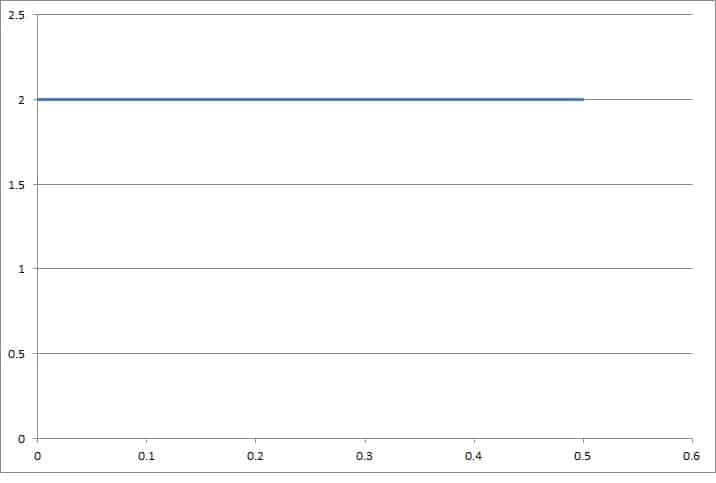# Can Probability Be Greater Than 1? (3 Things To Know)

Probability is used to calculate the chance that an event will happen.  There are certain rules for probability that help to keep this concept well-defined, including total probability.

So, can probability be greater than 1?  The probability of an event cannot be greater than 1.  A probability of 1 corresponds to a 100% chance (the event is guaranteed to happen).  If you calculate a probability greater than 1, check your assumptions and the independence of events.

Of course, a probability density function can have values greater than 1.  However, the total area under the curve still must equal 1.

In this article, we’ll talk about probability and why it can never be greater than 1.  We’ll also look at some examples where it is easy to make a mistake and conclude that probability is greater than 1.

Let’s get started.

## Can Probability Be Greater Than 1?

Probability cannot be greater than 1.  The probability of an event occurring can range from 0 to 1:

• A probability of 0 corresponds to a 0% chance (it is impossible for the event to happen).
• A probability of 1 corresponds to a 100% chance (the event is guaranteed to happen).

There is no such thing as “more than certain”, so no event has a probability greater than 1.

It is also true that no event can have a negative probability (this prevents the complement event from having a probability greater than 1).

Remember that the probability of an event and its complement must equal 1:

• P(A) + P(AC) = 1

The rules of probability restrict the range of values that probability can take on when we calculate the likelihood of events that involve AND or OR.

For example, to calculate the probability of events A and B occurring together, we multiply the probability of the two events.  That is:

• P(A and B) = P(A)*P(B)

Since P(A) and P(B) are both between 0 and 1, then P(A and B) will also be between 0 and 1.

To calculate the probability of events A or B occurring, we add the probability of the events and subtract the probability that they occur together.  That is:

• P(A or B) = P(A) + P(B) – P(A and B)

This equation makes it look like we might be able to get a probability greater than 1, since we are adding two probabilities.  However, subtracting P(A and B) ensures that we will always get a probability less than or equal to 1.

If you get a probability of more than 1 after calculating P(A or B), then you made a mistake.  It could be an arithmetic mistake, or it could be a mistaken assumption (for example, that two events are mutually exclusive and P(A and B) = 0).

Let’s take a look at an example to see how this mistake could happen.

### Example: Calculating P(A or B)

Let’s define the following events:

• A = the event that Microsoft stock goes up today
• B = the event that Google stock goes up today

You assign the following probabilities, based on your best estimate:

• P(A) = 0.6
• P(B) = 0.7

If we simply add these probabilities, we will get a value greater than 1.  However, this would be a mistake, since we cannot get a probability greater than 1.

So, events A and B are not mutually exclusive.  That is, they can both happen together.

This makes sense, since it is possible for both Microsoft and Google stock to increase on the same day.

In fact, since P(A) + P(B) = 0.6 + 0.7 = 1.3, we know that P(A and B) is at least 0.3, since we know that the total probability is less than or equal to 1:

• P(A or B) <= 1  [no probability can be greater than 1]
• P(A) + P(B) – P(A and B) <= 1  [formula for P(A or B)]
• 0.6 + 0.7 – P(A and B) <= 1  [substitute P(A) = 0.6 and P(B) = 0.7 from above]
• 1.3 – P(A and B) <= 1
• -P(A and B) <= -0.3
• P(A and B) >= 0.3  [reverse inequality symbol after dividing by -0.3]

This is a lower bound, since P(A and B) could be larger.

## Can Conditional Probability Be Greater Than 1?

Conditional probability cannot be greater than 1.  The conditional probability of an event is still a probability, and its value can be at most 1 (guaranteed to happen).

The conditional probability of event B occurring, given than event A occurs, is:

• P(B|A) = P(A and B) / P(A)

This equation might suggest that we can get a probability greater than 1, since we are dividing by P(A), which could be a decimal less than 1.

However, remember that event A occurring is always at least as likely as A and B occurring together.  In other words:

• P(A) >= P(A and B)

The two probabilities P(A) and P(A and B) can be equal in this scenario: when event B is guaranteed to happen if event A happens.

This is easier to see with a Venn diagram of the events. In this first diagram, there is some overlap between events A and B:Events A and B have some overlap, but A and B is not completely contained in A.

In this second diagram, event B (the small circle) is completely contained in the larger circle (event A), and so P(A and B) is less than P(A):Event B is completely contained in event A, and thus A and B is contained completely in A.

For example, let’s say that A is the event that we get at least 2 inches of rain today, and B is the event that we get at least 1 inch of rain today.

If event A happens (we get at least 2 inches of rain today), then event B automatically happens (since we also got more than 1 inch of rain today).  Thus P(A) = P(A and B) in this case.

If the circles were the exact same size and overlapped completely, then we would have P(A) = P(B) = P(A and B) = P(A or B).

If we start with the equation above, we can prove that our conditional probability is never greater than 1:

• P(A) >= P(A and B)  [based on the Venn diagram]
• P(A) / P(A) >= P(A and B) / P(A)  [divide by P(A) on both sides]
• 1 >= P(A and B) / P(A)
• 1 >= P(B|A)  [by definition of conditional probability, from above]

By similar reasoning, P(A|B) is also never greater than 1.

## Can Cumulative Probability Be Greater Than 1?

Cumulative probability cannot be greater than 1.  By definition, cumulative probability is counting the area under a probability density curve for a subset of the domain.

Since the total area under a PDF must equal 1, a cumulative probability cannot be greater than 1 (and must always be at least 0 – it can never be negative).

Remember that the cumulative density function (CDF) is found by integrating the probability density function (PDF) over an interval.

## Can Probability Density Be Greater Than 1?

A probability density function can be greater than 1 in some cases.  The only requirement is that the total probability (area under the curve, found by integrating the probability density function) is 1.

Here is a simple example.

### Example: Probability Density Function Greater Than 1 On Its Entire Domain

Consider the simple example of a uniform probability density function (one that always has the same value):

• f(x) = 2, for 0 <= x <= 0.5

The total area under this curve is easy to calculate, since the shape is a rectangle with a width 0.5 and height of 2:

• width*height = 0.5*2 = 1

So, the total area under this probability density function is 1.  It is never negative, but the probability is greater than 1 at every point on the interval [0, 0.5].This uniform probability density function has a value of 2 on the entire interval [0. 0.5], which means the area under the curve is 1 (total probability equals 1).

## Conclusion

Now you know that probability cannot be greater than 1.  You also know that certain probability density functions can have values greater than 1, as long as the total area under the curve is 1.

You might also want to check out my article on the difference between probability & statistics.